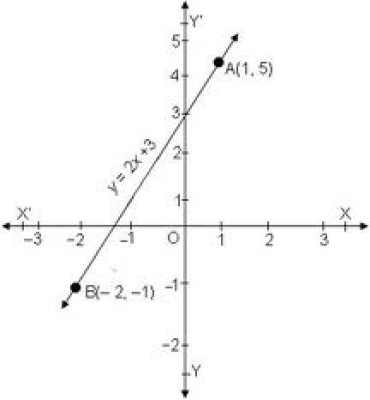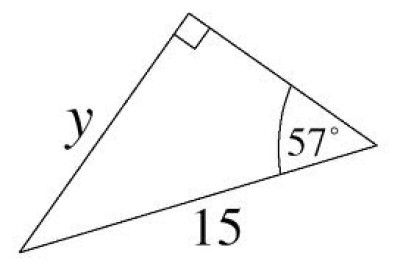List of public pages created with Protopage

# Home

## Calendars

### Calendar

• Sun October 7 - Lunchtime: Buy a new beanie hat
• Mon October 8 - 6pm: Go rollerblading

# Bookmarks

## Plain sticky notes

### Sticky note

Click 'edit' on the Bookmarks widget to add all of your favorite sites to your page for quick access

# Linear equations

## Plain sticky notes

### Sticky note

Linear grapghs are used to represent a group of data, they can be created through finding the coordinates/positions of each point by folowing the rule provided such as y=3x+8. Then each point is joined up to form a perfectly straight line. In order to plot the graph, you must work out the point through the rule, the rule is broken down in different steps: 1. Y can equl any number but is usually used as -3, -2, -1 0 1, 2 & 3 2. Then to find x, you multiply it by the number next to it in the rule, in this case it would be 3 3. you then add the end number to the number you have, and that it x 4. when all of the coordinates are found, you plot them on the graph and join them. The equation y=3x+8 has the results...

## Photos

### Example of a linear graph# Triganometry

## Plain sticky notes

### Sticky note

Triganommetry is used to find either missing sides or angles in triangles In order to answer triganometry questions, you must follow a formular that shows which buttons you must press on the calcuator, either sin, cos or tan, this formular is: o a o s h c h t a soh, shows that you would have a letter (usually x) or number on either the hypotonuse or the opposite line to the angle, then in order to find the opposite you enter (sin(ang)) x the hypotonuse lenght = opposite length. To find the hypotenuse, you do the same formular , but you multiply the sin by the opposite lenght which equals the hypotenuse. In order to find the angle, you must divide the opposite by the hypotenuse, then enter (cos-1) and press = . In order to solve other problems using triganometry, you use the same formular but using different basic formuars (soh cah toa) o = opposite length to the angle h = hypotenuse a = adjacent length to the angle

## Photos

### Can you answer this question?# Factorising

## Plain sticky notes

### Sticky note

in order to factorise an expression, you must identlfy the numerical similarities in the numbers given. An example of this would be the similarietes of 15 and 10, they are both multipuls of 5. There for in order to to solve: 15x+10 we identify the similarieties and show them by placing the 5 outside some bracets to show that it is a multipul and the most common factor of the to numbers of the nummbers inside, 5 x 3 equals 15 and 5 x 3 equals 10, therefor 15x+10 becomes 15x+10=5(3x)+5(2) then in order to finish the equation, we put the two bracets together into one so the answer reads: 15x+10=5(3x+2)

33x+18